Multi-Objective Antenna Optimization

For quite some time I'm optimizing antennas using genetic algorithms. I'm using the pgapack parallel genetic algorithm package originally by David Levine from Argonne National Laboratory which I'm maintaining. Longer than maintaining pgapack I'm developing a Python wrapper for pgapack called pgapy.

For the antenna simulation part I'm using Tim Molteno's PyNEC, a python wrapper for the Numerical Electromagnetics Code (NEC) version 2 written in C++ (aka NEC++) and wrapped for Python.

Using these packages I've written a small open source framework to optimize antennas called antenna-optimizer. This can use traditional genetic algorithm method with bit-strings as genes as well as a floating-point representation with operators suited for floating-point genes.

The parallel in pgapack tells us that the evaluation function of the genetic algorithm can be parallelized. When optimizing antennas we simulate each candidate parameters for an antenna using the antenna simulation of PyNEC. Antenna simulation is still (the original NEC code is from the 1980s and was conceived using punched cards for I/O) a CPU-intensive undertaking. So the fact that with pgapack we can run many simulations in parallel using the message passing interface (MPI) standard  is good news.

For pgapack – and also for pgapy – I've recently implemented some classic algorithms that have proven very useful over time:

• Differential Evolution , ,  is a very successful optimization algorithm for floating-point genes that is very interesting for electromagnetics problems
• The elitist Nondominated Sorting Genetic Algorithm NSGA-II  allows to optimize multiple objectives in a single run of the optimizer
• We can have constraints on the optimization using constraint functions that are minimized. For a solution to be valid, all constraints must be zero or negative. 

Traditionally with genetic algorithms only a single evaluation function, also called objective function is possible. With NSGA-II it is possible to have several objective functions. We call such an algorithm a multi-objective optimization algorithm.

For antenna simulation this means that we don't need to combine different antenna criteria like gain, forward/backward ratio, and standing wave ratio (VSWR) into a single evaluation function which I was using in antenna-optimizer, but instead we can specify them separately and leave the optimization to the genetic search.

With multiple objectives, however, typically when a solution is better in one objective, it can be worse in another objective and vice-versa. So we are searching for solutions that are strictly better than other solutions. A solution is said to dominate another solution when it is strictly better in one objective but not worse in any other objective. All solutions that fulfill this criterion are said to be pareto-optimal named after the italian scientist Vilfredo Pareto who first defined the concept of pareto optimality. All solutions that fulfill the pareto optimality criterion are said to lie on a pareto front. For two objectives the pareto front can be shown in a scatter-plot as we will see below.

Since pgapack follows a "mix and match" approach to genetic algorithms we can combine successful strategies for different parts of a genetic algorithm:

• We can use Differential Evolution just for the mutation/crossover part of the genetic algorithm
• We can combine this with the nondominated sorting replacement of NSGA-II
• We can define some of our objectives as constraints. For our problem it makes sense to only allow antennas that do not exceed a given standing-wave ratio. So we do not allow antennas with a VSWR > 1.8. The necessary constraint function is $S - 1.8 \le 0$ where $S$ is the voltage standing wave ratio (VSWR).

With this combination we can successfully compute antennas for the 70cm ham-radio band (430 MHz - 440 MHz). The antenna uses what we call a folded dipole (the thing with the rounded corners) and a straight element. The measures in the figure represent the lenghts optimized by the genetic algorithm. The two dots in the middle of the folded dipole element represent the point where the antenna feed-line is connected.A first example simulates antenna parameters for the lowest, the highest and the medium frequency. The gain and forward/backward ratio are computed for the medium frequency only: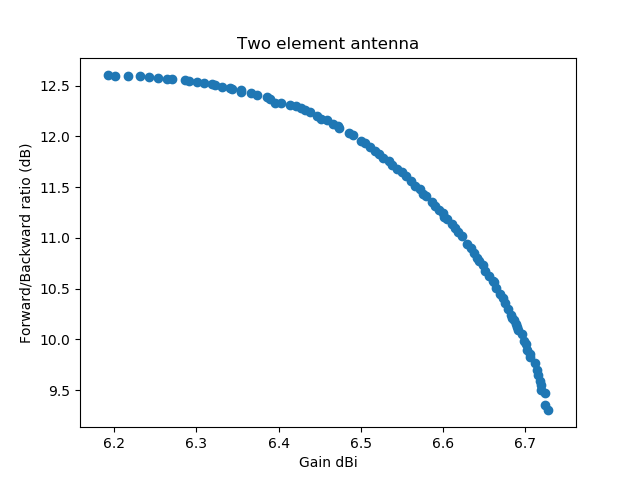In this graph (a scatter plot) the first objective (the gain) is graphed against the second objective, the forward/backward ratio. All numbers are taken from the medium frequency. Each dot represents a simulated antenna. All antennas have a VSWR lower than 1.8 on the minimum, medium, and maximum frequency.

With this success I was experimenting with different settings of the Differential Evolution parameters. It is well-known that Differential Evolution performance on decomposable problems is better with a low crossover-rate, while it is better on non-decomposable problems with a high crossover rate. A decomposable problem is one where the different dimensions can be optimized separately, this was first observed by Salomon in 1996 . I had been using a crossover-rate of 0.2 and my hope was that the optimization would be better and faster with a higher crossover rate. The experiment below uses a crossover-rate of 0.9.

In addition I was experimenting with dither: Differential Evolution allows to randomly change the scale-factor $F$, by which the difference of two vectors is multiplied slightly for each generated variation. In the first implementation I was setting dither to 0, now I had a dither of 0.2. Imagine my surprise when with these settings I found a completely different Pareto front for the solution: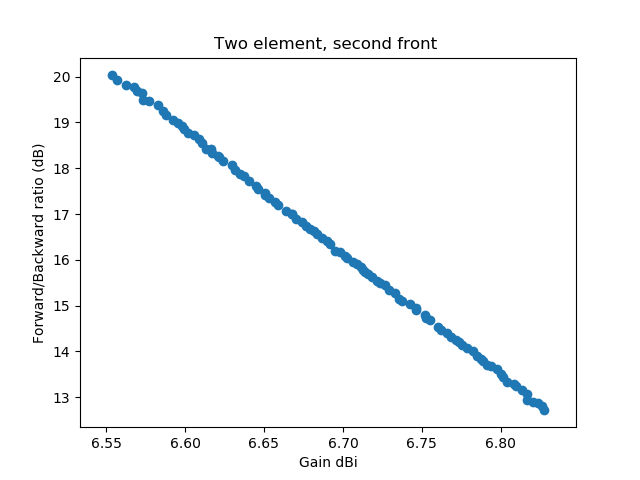To make it easier to see that the second discovered front completely dominates the front that was first discovered, I've plotted the two fronts into a single graph: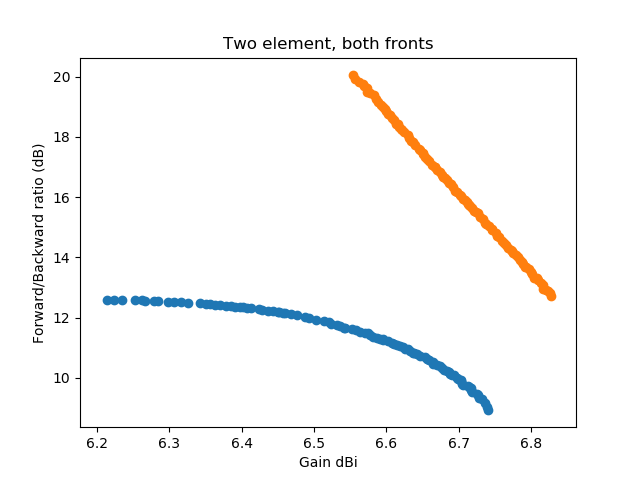Now since the second discovered front looks too good to be true (over the whole frequency range) for a two-element antenna, lets take a look what is happening here. First we show the orientation of the antenna and the computed gain pattern for one of the antennas from the middle of the lower front: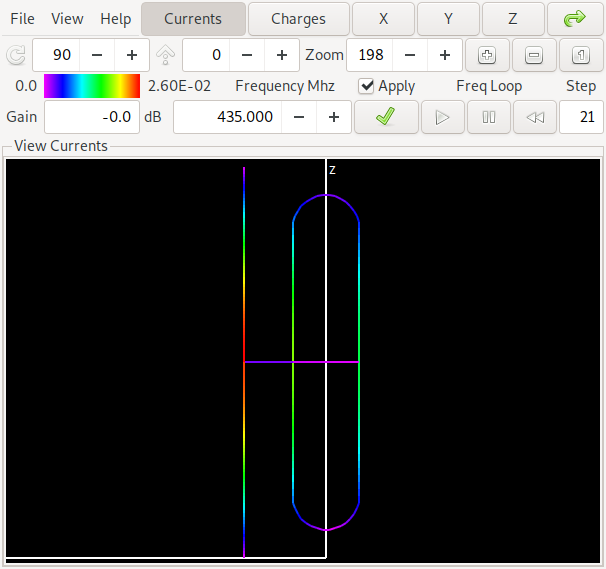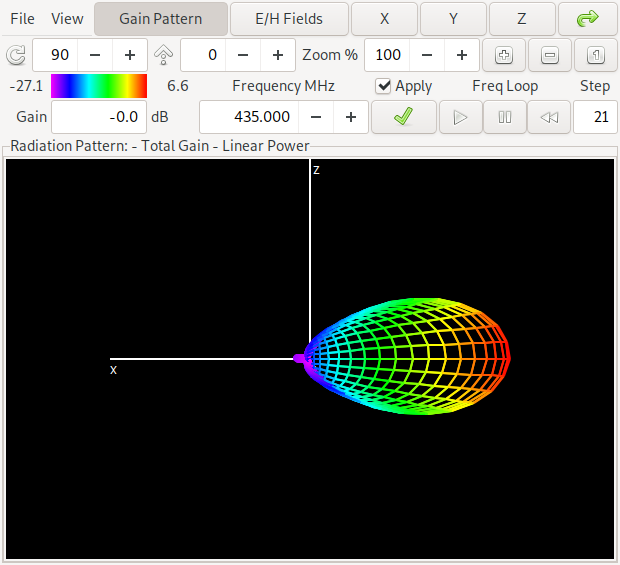The antenna has – as already indicated in the pareto-front graphics – a gain of about 6.6 dBi and a forward/backward ratio of about 11 dB in the middle of the band at 435 MHz. The colors on the antenna denote the currents on the antenna structure. If you want to look at this yourself, here is a link to the NEC input file for antenna 1

Now lets compare this with one of the antennas of the "orange front", where we get a lot better values: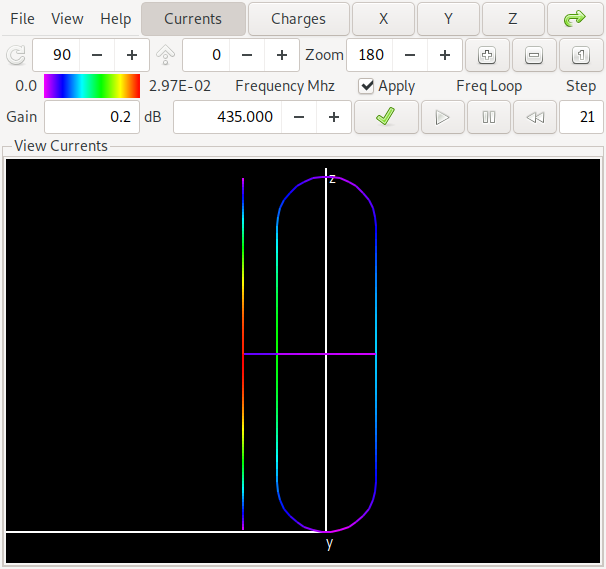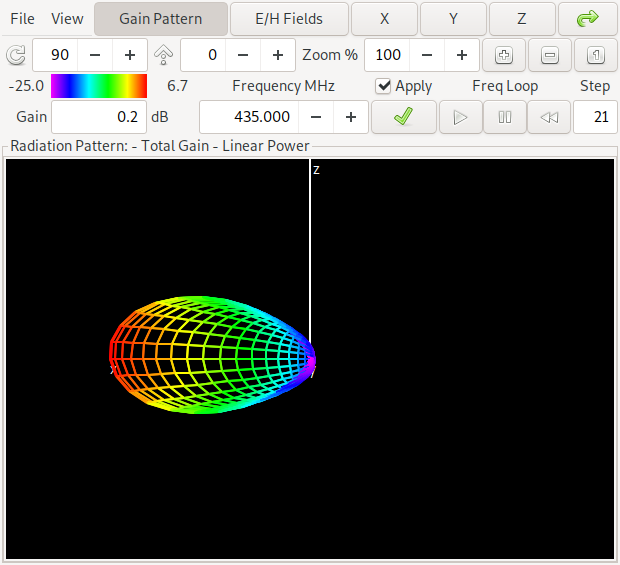This antenna is in the middle of the pareto front above and has a gain of about 6.7 dBi and a forward/backward ratio of about 16 dB in the middle of the band at 435 MHz. Can you spot the difference to the first antenna? Yes: The maximum gain is in the opposite direction of the first antenna. We say that for the first antenna the straight element acts as a reflector while for the second antenna it acts as a director. If you want to look at this yourself, here is a link to the NEC input file for antenna 2

Now we look at the frequence plot of gain and forward/backward ratio of the antennas, the plot for the first antenna (with the reflector element) is on the left, while the plot for the antenna with the director element is on the right.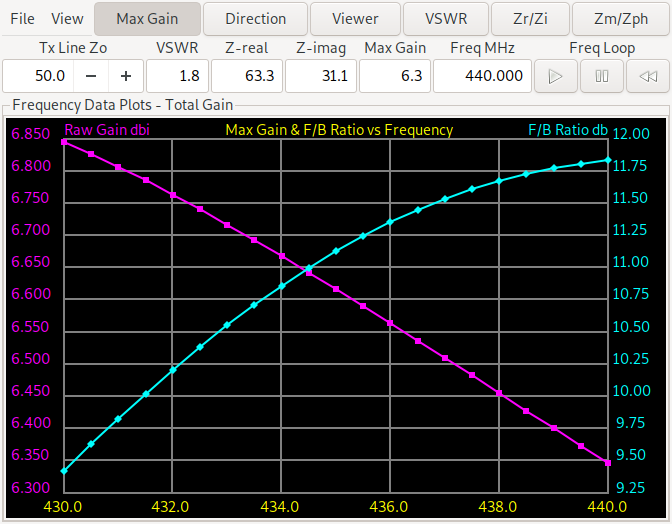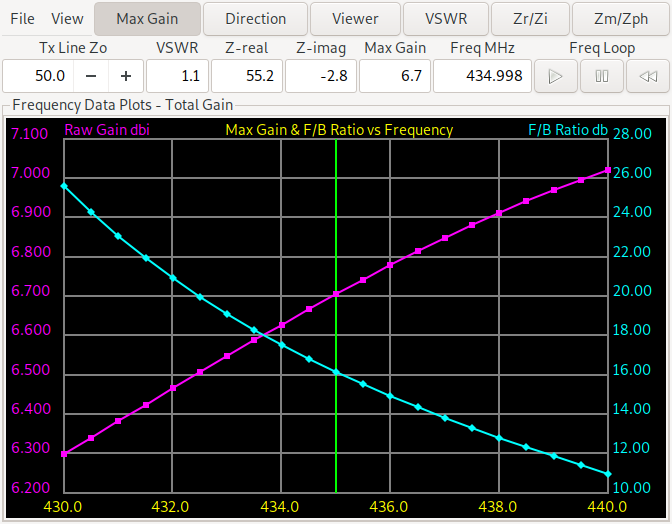We see that the forward/backward ratio of the director antenna ranges from more than 10 dB to more than 25 dB while the reflector design ranges from 9.3 dB to 11.75 dB. For the minimum gain the reflector design is slightly better (from 6.35-6.85 dBi vs. 6.3-7.05 dBi). So this needs further experiments. When forcing a reflector design and changing the evaluation function to return the minimum gain and F/B ratio over the three (start, middle, end) frequencies we get: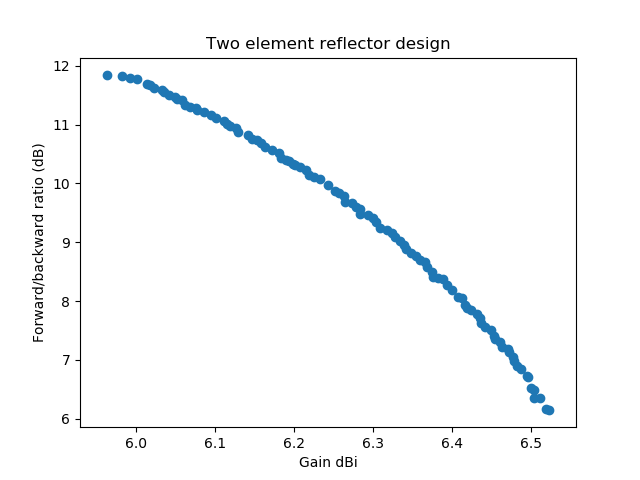The same for a director design (also with the minimum gain and F/B ratio over the three frequencies start, middle, end) we get: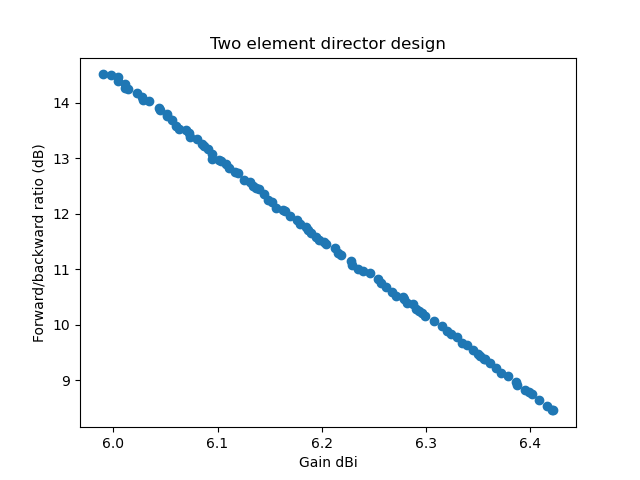With these result, the sweet spot for an antenna to build is probably at or above 10 dB F/B ratio and a gain of about 6.2 dBi. Going for some 1/10 dBi more gain and sacrificing several dB of F/B ratio doesn't seem sensible. Comparing the director vs. reflector design we notice (contrary to at least my intuition) that the director design has a better F/B ratio over the whole frequency range. If, however the antenna is to be used for relay operation, where the sending frequency (the relay input) is in the lower half of the frequency range and the relay output (the receiving frequency) is in the upper half, we will probably chose a reflector design because there the gain is higher when sending and the F/B ratio is higher when receiving (compare the two earlier gain and F/B ratio plots).

Also note that the optimization algorithm has a hard time finding the director solutions at all. Only in one of a handful experiments I was able to obtain the pareto front plotted above. The design is more narrowband than the reflector design and the algorithm often converges to a local optimimum. The higher difference in gain and F/B range of the director design also tells us that it will be harder to build: Not getting the dimensions exactly right will probably not reach the predicted simulation results. The reflector design is a little more tolerant in this regard.

  MPI: A message-passing interface standard, version 4.0. Standard, Message Passing Interface Forum, June 2021.
  Rainer Storn and Kenneth Price. Differential evolution – a simple and efficient adaptive scheme for global optimization over continuous spaces. Technical Report TR-95-012, International Computer Science Institute (ICSI), March 1995.
  Rainer Storn and Kenneth Price. Differential evolution – a simple and efficient heuristic for global optimization over continuous spaces. Journal of Global Optimization, 11(4):341–359, December 1997.
  Kenneth V. Price, Rainer M. Storn, and Jouni A. Lampinen. Differential Evolution: A Practical Approach to Global Optimization. Springer, Berlin, Heidelberg, 2005.
  Kalyanmoy Deb, Amrit Pratap, Sameer Agarwal, and T. Meyarivan. A fast and elitist multiobjective genetic algorithm: NSGA-II. IEEE Transactions on Evolutionary Computation, 6(2):182–197, April 2002.
  Kalyanmoy Deb. An efficient constraint handling method for genetic algorithms. Computer Methods in Applied Mechanics and Engineering, 186(2–4):311–338, June 2000.
  Ralf Salomon. Re-evaluating genetic algorithm performance under coordinate rotation of benchmark functions. a survey of some theoretical and practical aspects of genetic algorithms. Biosystems, 39(3):263–278, 1996.

Impedance Transformation on a Transmission Line

As a ham-radio operator one is confronted with the challenge to connect an antenna to a radio via a transmission line. Now often the impedance of the antenna is different from the impedance used by the radio and the transmission-line between antenna and radio. The impedance of radio and a typical coax transmission line is usually 50Ω in ham radio applications. The impedance of the antenna varies and depends on the technology and environmental factors.

A transmission line transforms the impedance of the antenna depending on how long it is. A well-known formula for this is given in Chipman  p.134 formula 7.15:

\begin{equation*} \frac{Z_d}{Z_0} = \frac{e^{\gamma d}(Z_l/Z_0 + 1) + e^{-\gamma d}(Z_l/Z_0 - 1)} {e^{\gamma d}(Z_l/Z_0 + 1) - e^{-\gamma d}(Z_l/Z_0 - 1)} \end{equation*}

In this formula $Z_d$ is the impedance at distance $d$ from the load, $Z_l$ is the impedance at the load (e.g. at the antenna) and $Z_0$ is the characteristic impedance of the transmission line and the radio, typically 50Ω, $\gamma$ is the complex transmission coefficient which can be split into the real- and imaginary parts, where $\alpha$ is the attenuation in Nepers/m and $\beta$ is the phase constant, $j$ is imaginary unit (often written as $i$ but in electrical engineering it is most often denoted as $j$):

\begin{equation*} \gamma = \alpha + j \cdot \beta \end{equation*}

It is sometimes claimed that a cable can improve the standing-wave ratio at the radio because it transforms the impedance of the cable. This is only true if the cable has a characteristic impedance different from the input/output impedance of the radio (if we asume that the cable is lossless which holds in many cases for short cables) which I'm going to show in the following.

In the lossless case $\alpha$ in the formula above is 0. We can express $\beta$ in terms of frequency and, finally, the wavelength $\lambda$:

\begin{equation*} \beta = \frac{2\pi f}{c * \mathbb{VF}} \end{equation*}

and:

\begin{equation*} \lambda = \frac{c}{f} \end{equation*}

The frequency $f$ is given in Hz, $c$ is speed of light and $\mathbb{VF}$ is the velocity factor of the transmission line. When we express the distance from the load $d$ in Chipman's formula as $l_\lambda$ a multiple of of $\lambda$, $f$, $c$, and $\mathbb{VF}$ cancel and for the lossless case we get:

\begin{equation*} \frac{Z_d}{Z_0} = \frac{ e^{ 2\pi l_\lambda j}(Z_l/Z_0 + 1) + e^{-2\pi l_\lambda j}(Z_l/Z_0 - 1) } { e^{ 2\pi l_\lambda j}(Z_l/Z_0 + 1) - e^{-2\pi l_\lambda j}(Z_l/Z_0 - 1) } \end{equation*}

The complex reflection coefficient $\rho$ is given as (e.g. in Chipman  7.9 p.128):

\begin{equation*} \rho = \frac{Z - Z_0}{Z + Z_0} \end{equation*}

where $Z$ is the impedance at the point on the line we want to know the reflection coefficient. From this we can compute the voltage standing wave ratio (see e.g. Chipman  8.21 p.165) which I'm calling here $S$ for convenience:

\begin{equation*} S = \frac{1+|\rho|}{1-|\rho|} \end{equation*}

A simple Python program (python is very convenient for computations like this because it supports complex numbers out of the box and is free) for computing all this might look like:

from math import e, pi
def impedance (z_load, l_lambda, z0 = 50.0):
ex = 2j * pi * l_lambda
lp = e **  (ex) * (zl + 1)
lm = e ** (-ex) * (zl - 1)
return z0 * (lp + lm) / (lp - lm)

def vswr (z, z0 = 50.0):
absrho = abs ((z - z0) / (z + z0))
return (1 + absrho) / (1 - absrho)

If we compute several points for this, e.g., with an antenna that has 72Ω and various multiples of $\lambda$ we get the same value for the standing wave ratio:

$l_{\lambda}$ $Z_d$ $S$
0 72+0j 1.44
1/8 46.85-17.46j 1.44
3/8 46.85+17.46j 1.44
1/4 34.72-1.59j 1.44
1/2 72+0j 1.44

To prove that the standing wave ratio is always the same, no matter how long the transmission line is, is left as an exercise to the reader, a hint: It is enough to prove that the absolute value of $\rho$ stays the same.

Now the more interesting case is when we take cable losses into account. I've written a piece of software that can model a coax line from manufacturer data, an idea that was published long ago by Frank Witt, AI1H . The implementation is part of my open source antenna-optimizer project and features a command-line utility called coaxmodel. With it you can compute the input impedance (and standing wave ratio) for a real cable. The implementation already contains models of some cables but it is easy to add more (see at the end of coaxmodel.py). In addition it allows you to compute a stub match: Adding a parallel piece of transmission line in parallel to the feed line at a certain distance from the load (this piece of line is called a stub) will transform the impedance in a way that the generator (the transceiver) will see a 50Ω match. It would compute for the example given above (only a subset of the output is given here, try it yourself):

% coaxmodel -z 72 -f 435e6 -l .057 match
0.06 m at 435.00 MHz with 100 W applied
Input impedance 46.857 -17.433j Ω
VSWR at input 1.439
Inductive stub with open circuit at end:
Stub attached 0.06246 m from load
Stub length 0.20224 m
Resulting impedance 50.00 -0.00j

This tells us that a 5.7cm transmission line will transform the 72Ω impedance at the load to a 46.86-17.43jΩ impedance at the input end of the feed line. It is also visible that the standing wave ratio at the input has improved very slightly due to losses in the cable and that at this length the difference is negligible.

Attaching a 20.2cm piece of 50Ω wire with an open circuit at the end in parallel to the feed wire at a distance of 6.2cm from the load will transform the impedance to 50Ω resulting in a standing wave ratio of 1:1.

  (1, 2, 3) Robert A. Chipman. Theory and Problems of Transmission Lines. Schaums Outline. McGraw-Hill, 1968.
  Frank Witt. Transmission line properties from manufacturer’s data. In Straw , pages 179–183.
  R. Dean Straw, editor. The ARRL Antenna Compendium, volume 6. American Radio Relay League (ARRL), 1999.

Enabling FEL-Mode on Orange-Pi Zero for Flashing U-Boot to NOR-Flash

The Orange-Pi Zero is a popular open hardware single-board computer that can run Linux. It is well supported by current Linux kernels and the U-Boot bootloader.

Recent boards feature a NOR-Flash that can be used to store the U-Boot bootloader. You build U-Boot from source with the commands:

make orangepi_zero_defconfig
make

And then using the sunxi-tools (Debian Linux has these tools as a package for two releases or so) you can flash the bootloader into the NOR Flash. You first connect a Micro-USB cable from the Orange-Pi USB-OTG port (thats the small µ-USB on the board) to a computer (preferrably running Debian Linux, for other systems you're on your own). The Orange-Pi should not have a SD-card inserted. In my experiments the power supplied via USB to the Orange-Pi was enough to flash the boatloader to NOR flash using the command:

sunxi-fel -v -p spiflash-write 0 u-boot-sunxi-with-spl.bin

The file u-boot-sunxi-with-spl.bin was just built with the commands above. So far everything is documented on the sunxi.org Orange-Pi Zero site. Once you have flashed the bootloader, though, the Orange-Pi will no longer boot into the FEL mode needed for the above command to work, instead it will start U-Boot from NOR flash. If you ever want to flash a new version of U-Boot you need to start the Orange-Pi in FEL mode again. To get the Orange-Pi into FEL mode again, some methods are also documented on the sunxi.org site. Unfortunately the easiest method: Setting the RECOVERY pin to ground which is supported by many boards with a jumper is not so easy on the Orange-Pi Zero because the pin is connected to a pull-up resistor (R 123) without any jumper attached. Fortunately the resistor is easily accessible on the board, I've marked the side of R 123 on the board with the right red arrow in the picture.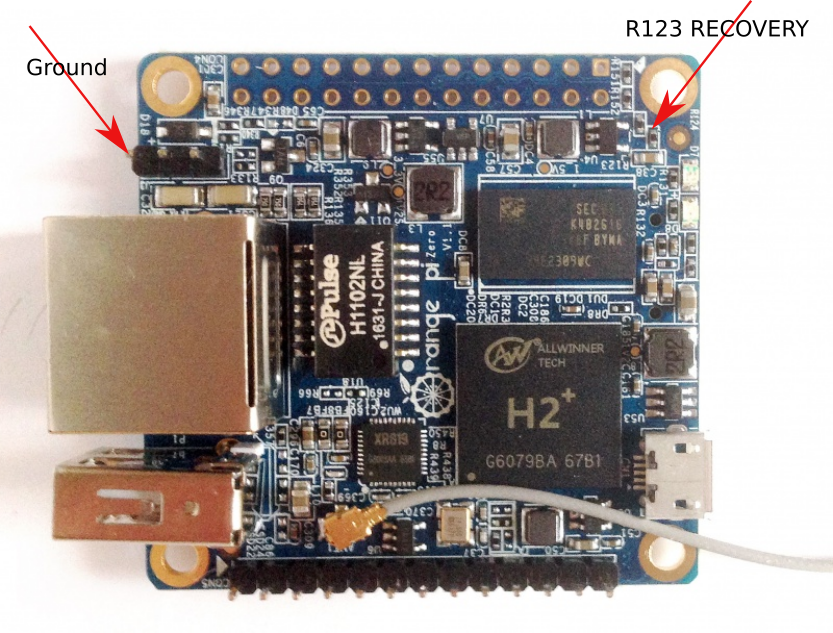This has to be connected to ground. I'm using the ground on the debug serial port (marked with the left red arrow) because all other ground connection are adjacent to a +5V pin and if you accidentally manage to connect +5V to something other on the board you could destroy it. So the ground pin on the debug serial port is the safest choice. You connect ground to the R 123 pin, both marked with red arrows, and then you power up the board (e.g. by connecting the OTG-USB). You can verify the board is really in FEL mode with the command:

sunxi-fel ver

This prints version information for the board and will issue an error message if FEL mode was not successfully entered. Once in FEL mode you can re-flash the boot loader if needed.

Success-message from the command above:

AWUSBFEX soc=00001680(H3) 00000001 ver=0001 44 08 scratchpad=00007e00 00000000 00000000

Error message if FEL mode was not entered:

Q-Factor of a Coax Resonator

When studying transmission line theory recently for modelling transmission lines in my antenna-optimizer project, I stumbled upon a formula of the Quality (Q) factor of a coax resonator by Frank Witt :

\begin{equation*} \frac{2.774 F_0}{A \cdot \mathbb{VF}} \end{equation*}

In this formula $F_0$ is the frequency of the resonator in MHz, $A$ is the loss in dB per 100 ft, and VF is the velocity factor of the cable. The formula is for a $\frac{\lambda}{4}$ resonator. I wondered about this because, since the loss in that formula is a logarithmic quantity (dB) the computation of the Q-factor should involve exponentiation.

When using the stored energy definition of the Q-factor from Wikipedia, we get:

\begin{equation*} 2 \pi \frac{E_s}{E_d} \end{equation*}

where $E_s$ is the stored energy and $E_d$ is the energy dissipated per cycle.

We know that the loss factor of a cable in dB involves power loss. If the loss in dB per 100m (we're using metric units) is $a$ we have for the loss in dB:

\begin{equation*} \frac{a \cdot l}{100} \end{equation*}

where $l$ is the length im meter. For a $\frac{\lambda}{4}$ resonator we get:

\begin{equation*} \frac{a \cdot \mathbb{VF}\lambda}{4\cdot 100} \end{equation*}

To compute the fraction of the power lost (instead of the logarithm of the fraction in dB) when transmitting we get

\begin{equation*} 1 - 10^{-\frac{a \cdot \mathbb{VF}\lambda} {4 \cdot 100 \cdot 10}} \end{equation*}

Replacing

\begin{equation*} \lambda = \frac{c}{f_0} \end{equation*}

into the formula where $c$ is the speed of light and $f_0$ is the resonance frequency in Hz we get

\begin{equation*} 1 - 10^{-\frac{a \cdot c\cdot\mathbb{VF}} {4 \cdot 100\cdot 10\cdot f_0}} \end{equation*}

Going back to the Wikipedia formula which involves energies to get an energy ratio from a power ratio we would have to integrate – but since this would yield the same result for the ratio we instead do a handwaving integration here: We need to get the travelled distance straight: The Wikipedia definition involves one whole period $\lambda$, not $\frac{\lambda}{4}$. And the Q-factor is the ration of the stored power to the lost power. So we have:

\begin{equation*} Q = \frac{2\pi}{1 - 10^{-\frac{a \cdot c\cdot\mathbb{VF}}{1000\cdot f_0}}} \end{equation*}

Note that this makes the resonator $Q$ independent of the type of resonator, be it a $\frac{\lambda}{4}$ or $\frac{\lambda}{2}$ resonator.

When plotting Q-factors for some shortwave frequencies (they're in MHz in the figure) against the loss in dB we see that the formula derived above is fairly close to the approximation formula from Witt .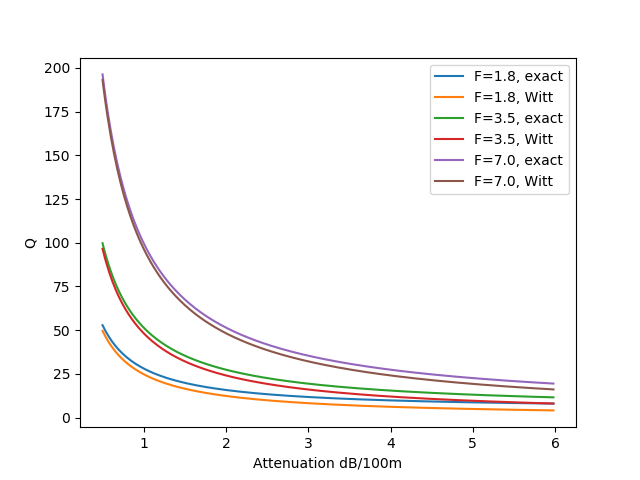When plotting Q-factor against frequency (also in the shortwave range) for certain common cable types we also see that the error is not too high, especially for higher frequencies.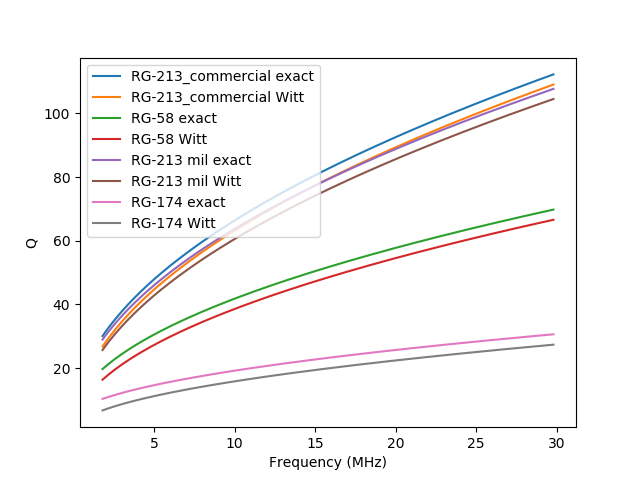The relative errors can also be plotted, also for some common cable types over the whole shortwave range. We see that the error is quite high for the low frequency range and is higher for the more lossy cable types like RG174.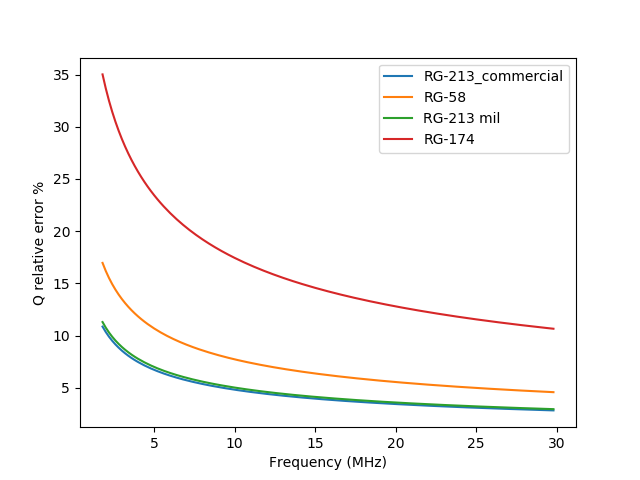So we see that the formula is probably some approximation and is fairly accurate for higher frequencies and low loss. Now the question was open: Where does this formula come from and what is the magic constant that obviously lumps all physical constants into a magic number?

When studying transmission line theory I also stumbled over an old book on the subject which once was a university textbook . On p. 222 Chipman derives an approximate formula for $Q$ with a simplifying assumption that $\alpha$ (the attenuation coefficient in nepers per meter) is small. Chipman's approximate formula is:

\begin{equation*} Q \approx\frac{\beta_r}{2\alpha_r} \end{equation*}

where $\alpha$ is the attenuation coefficient in nepers per meter and $\beta$ is the phase factor of the line in radians per meter. The subscript $r$ stands for resonance. We can write

\begin{equation*} \lambda=\frac{c\mathbb{VF}}{f_0} \end{equation*}

and

\begin{equation*} \beta_r=\frac{2\pi}{\lambda} \end{equation*}

and Witts loss $A$ per 100ft can be written (converting nepers to dB) as

\begin{equation*} A=\frac{20\cdot 100\alpha_r}{3.2808\cdot log_e 10} \end{equation*}

Where the constant 3.2808 is the conversion factor m/ft. Solving for $\alpha_r$ and replacing $\lambda$ and $\alpha_r$ into Chipman's formula we get:

\begin{equation*} Q \approx\frac{2\cdot 2000\pi f_0} {2 c \mathbb{VF}\cdot A \cdot 3.2808\cdot log_e 10} \approx\frac{2.774 F_0}{A \mathbb{VF}} \end{equation*}

Where the final $F_0$ is $f_0$ in MHz.

We see that the low-$\alpha$ asumption of Chipman's (and Witt's) formula holds for higher frequencies and low loss. We've already seen that the approximation if fairly good for low-loss cables: $\alpha$ in nepers per meter is a constant factor from the loss-figure in dB (be it per 100m or per 100ft). That it gets better with higher frequencies is because the loss of a cable typically increases with the square-root of the frequency while $\lambda$ decreases inverse proportionally with frequency. So, e.g., a $\frac{\lambda}{4}$ resonator has higher loss at a lower frequency.

  (1, 2) Frank Witt. The coaxial resonator match. In Gerald L. (Jerry) Hall, editor, The ARRL Antenna Compendium, volume 2, pages 110-118. American Radio Relay League (ARRL), 1989.
  Robert A. Chipman. Theory and Problems of Transmission Lines. Schaums Outline. McGraw-Hill, 1968.

In two older posts in this blog, one about a Second SPI chipselect for Orange-Pi Zero, one about a Hitachi HD44780 text display under Linux, I talked about trying to get changes into the Linux Kernel.

In case of the Orange-Pi it was a bug-fix to the SPI-Driver of the Allwinner sun6i architecture. Thanks at this point to the author Mirko of that patch who let me submit the patch in his name. The patch is in the kernel since shortly before 5.13. Since it has been marked as a bug-fix, it was backported to various stable series of the kernel as far back as the 4.4 stable series.

In case of the Hitachi display it was a documentation update that should allow people to find out how to connect such a display with the necessary device tree magic but without any software change. This patch has finally been accepted into the Linux Kernel in time for the 5.15 release.

Modding a PC Power Supply

WARNING: In the following I'm going to describe how to modify a power supply. Power supplies contain high voltages – even after removing the power plug they can still have high charges in capacitors. In some countries devices with mains power may be modified only by or under supervision of certified persons. So you should know what you're doing and be authorized to modify a power supply.

PC power supplies are cheap and readily available but the tend to produce the correct voltages only when the drawn current from all different voltages are within the minimum/maximum specs of the power supply. Drawing high current (but within the specs of the power supply) only from a single voltage lets this voltage drop below specification.

One example is the heat-bed of my 3D-printer: It used to have a PC power supply for the heat bed. The heat bed draws about 12A but only from the 12V line. The result was a voltage of about 10V when the heat-bed was heating.

Another use-case is the supply of several ARM based single-board computers (e.g. Raspberry-Pi or Orange-Pi) from a single 5V line. When using a PC-Power supply for this (without drawing current from the 12V lines) the nominal 5V voltage may drop below a value where the single-board computer works reliably.

A third example is the use of a power supply for powering the radio of a ham-radio operator: These radios typically don't output full power when powered with 12V or less, they typically need 13.6-13.8V for full power operation.

In all these cases a modification of the power supply that keeps the chosen voltage stable or even allows to modify the chosen voltage slightly (from 12V to 13.8V for hamradio operation) would be nice. How can we achieve that?

Many PC power supplies are based on the power regulator integrated circuits TL494 or KA7500 (they are pin-compatible). If you have one of those they can usually be modified for the purposes outlined above.

One schematic details of those supplies is a feedback-circuit that feeds back the 5V and the 12V voltage to the regulator IC. You can find a lot of power supply schematics on Dan's PC power supply page. Take the second of the TL494 or KA7500 based supplies, it has several resistors in parallel from pin 1 of the TL494 to ground, a 27kΩ-resistor from 12V to pin 1 and a 4.7kΩ-resistor from 5V to the same pin.

We can modify the voltage regulation by changing the feedback pins. Note that every power supply usually has different resistors to ground and different feedback resistors from 5V and 12V. As an example we replace the two feedback resistors to make the power supply provide stable 5V without caring for the 12V supply.

WARNING: When modifying a power supply for a stable 5V or 12V source, the other voltages will no longer be stable and may become too high for use in a PC. You should never use such a modified power supply in a PC.

So the first step is to identify the two resistors in the power supply. Once found we verify that the side of the resistor not connected to pin 1 of the regulator IC has 0Ω to the correct (5V or 12V) power supply output. We unsolder both resistors. Now before computing the new resistor to be placed between 5V output and pin 1 we measure the resistance between pin 1 and ground: Because we have now unsoldered the two resistors to 12V and 5V the resistor can be measured. It is good to be sure that the measured resistance matches the computed resistance from the three resistors connected in parallel: In the example we have 100kΩ, 390kΩ, and 10kΩ in parallel which should measure as

\begin{equation*} \frac{1}{\frac{1}{100000}+\frac{1}{390000}+\frac{1}{10000}} = 8883.83 \end{equation*}

When recently modifying a power supply the resistors to ground were 470kΩ, 100kΩ, and what I thought was 8.9kΩ: I interpreted the colors of the last resistor as grey-white-black-brown-brown. When measuring the three parallel resistors I measured it as 4.61kΩ instead of the expected 8033Ω. It turns out (after viewing the resistor in sunlight) that what I had interpreted as grey was really yellow-ish. So the resistor was really a 4.9kΩ resistor and the computed resulting resistance was 4625Ω.

To make the power supply regulate only for 5V we connect a new resistor from pin 1 of the regulator IC to 5V and leave the 12V feedback line unconnected. But how do we chose the new resistor? To find out we need to solve a set of equations: We know from Ohms law the relation of the voltages, resistors, and currents. We know from Kirchhoff that the current through the 12V feedback resistor and the current through the 5V feedback resistor must add up to the current through the resistors to ground. These relationships are given in this maxima spreadsheet.

We compute the reference voltage V_ref at pin 1 when both, the resistor from 5V and from 12V are connected. Then we chose the new resistor to 5V so that the voltage on pin1 stays the same.

For the example in Dan's second schematic we get 1800Ω for R_new. For the power supply I recently modified I've already given the resistors to ground. The resistor to 12V was 39kΩ and the resistor to 5V was 9.1kΩ. The resulting R_new for that power supply was 4865Ω which I realized by connecting a 4.7kΩ and a 150Ω resistor in series which was close enought to get a good 5V output. The computation is given as the second example in the spreadsheet.

A word of caution: When modifying the 5V power regulation or modifying a power supply for higher voltages (more than 12V) you should be aware that most PC power supplies have capacitors rated for 16V in the 12V output circuit. So when drawing high currents from the power supply modified for 5V the voltage on the 12V line may become too high for these capacitors. To be on the safe side the capacitors should be changed to types rated for 25V.

When modifying the 12V supply we use basically the same procedure, except that we now connect the 12V feedback resistor and leave the 5V feedback open. How to compute the resistor for the 12V feedback line is left as an exercise.

I recently have provided a customer with a link to a firewall image (using the Turris MOX router with a variant of OpenWRT) hosted on my own webserver. The image included keys material for an OpenVPN connection. The image file was in a hidden directory on my projects webserver. I monitored closely if there would be any downloads besides the one I expected from my customer.

I am aware providing key material via an unsecured channel is not the best security practice. And in the end I had to revoke the VPN key material in the image and provide my customer with a new key via a secure channel.

Now I said I monitored the downloads. About an hour (!) after my customer downloaded the image (at 21/Mar/2021:17:35:50 to be precise), it was accessed from another IP:

77.74.177.4 - - [21/Mar/2021:18:43:51 +0100] "GET /turris-image-XXXXXXX.zip HTTP/1.1" 200 77244886 "-" "Mozilla/5.0 (Windows NT 10.0; Win64; x64) AppleWebKit/537.36 (KHTML, like Gecko) Chrome/81.0.4044.92 Safari/537.36"

Looking up this IP via whois yields:

> whois 77.74.177.4
% This is the RIPE Database query service.
...
netname:        KL-NET3
descr:          Kaspersky Lab Internet
country:        RU
...
source:         RIPE
organisation:   ORG-KL28-RIPE
org-name:       Kaspersky Lab AO
country:        RU

My customer is using Kaspersky antivirus software. So the link was probably leaked to Kaspersky via the installed software. On the one hand it may well be that the purpose of Kaspersky downloading that link is a benign service (they may scan things for viruses) but in my case it means that non-public information was leaked. On the other hand it may well be that information gleaned that way is used for other purposes, too – we do not know.

Interaction of libvirt and AppArmor

I'm teaching at the University of Applied Science Burgenland in Eisenstadt (Austria). We recently had a lab (which took place in the lab in Eisenstadt but students were working from home due to Covid reasons) where the task is to set everything up for virtualisation and then live-migrate a running virtual machine to another server using libvirt (we're using the command-line with virsh).

For just one group out of several – with identical initial Debian installations, migration failed with an error message. The migration command was:

virsh -c qemu+ssh://root@primary/system migrate --live --unsafe \
debian-1 qemu+ssh://root@secondary/system

For the lab we're using NFS because setting up a more advanced filesystem would take too much time, that's why we're using the --unsafe option. The following error message resulted (error message broken to several lines, this was all in a single line):

error: internal error: Process exited prior to exec:
libvirt:  error : unable to set AppArmor profile
'libvirt-d22db7ca-50ca-43bd-b6da-1ccecf5a83e7' for '/usr/bin/kvm':
No such file or directory

It turned out that this group had managed to fill up the /var partition with logfiles but after cleanup this still did produce the same message. So the hunch here is that some files that AppArmor and/or libvirt create dynamically could not be created and that was the reason why this failed. It also turned out that some AppArmor files that were correctly installed on the first machine were missing on the second.

Trying to reinstall AppArmor and related files using apt-get with the --reinstall option did not work, the missing config files in /etc/apparmor.d were not re-created. So removing the packages with the purge command (which removes all config files) and then reinstalling everything fixed the installed AppArmor files and made the migration finally work. I have no idea which files were missing.

When googling for the error message above I found a debian bug-report Where one of the dynamically generated files in /etc/apparmor.d/libvirt was zero length. This, however was not the problem in our case but indicates that AppArmor isn't very good at checking errors when a filesystem is full. So there are probably other files that are dynamically generated that were the problem in our case.

The following sequence of deinstall and reinstall commands fixed the problem in our case, note that just removing files as in the debian bug-report did not fix the issue in our case:

dpkg --purge apparmor-utils apparmor-profiles
dpkg --purge apparmor
rm -rf /var/cache/apparmor
apt-get install apparmor apparmor-utils apparmor-profiles
dpkg --purge libvirt-daemon-system
apt-get install libvirt-daemon-system
systemctl restart libvirtd.service
systemctl restart virtlogd.service
systemctl restart virtlogd.socket

I'm not sure restarting the services is really necessary but there was another issue that libvirt could not connect to the virtlog socket and this was fixed by restarting the virtlog.{service,socket}.

Dynamic DNS with the bind DNS server

The popular DNS server bind allows to have a configuration that enables clients to change DNS entries remotely. Since some of the public dynamic DNS services have moved to a pay-only subscription model and since I'm running my own mail and web server at a hosting site I was searching for a way to roll my own dynamic DNS service. This already is back some years now but since the Howto I used at the time seems to be gone (at least from my google bubble) I'm documenting here how it was done.

I'm running this on a Debian buster server at the time of this writing, so if you're on a different system some details may change. I'm calling the domain for the dynamic services dyn.example.com in the following.

The top-level config file of bind needs to include an additional config file for the dynamic domain. In my configuration this file is named.conf.handedited. In this file you need an entry for each dynamic DNS client as follows:

zone "dyn.example.com" {
type master;
allow-transfer {none;};
file "/etc/bind/slave/pri.dyn.example.com";
update-policy {
grant h1.dyn.example.com. name h1.dyn.example.com. A TXT;
grant h2.dyn.example.com. name h2.dyn.example.com. A TXT;
[...]
};
};

In this example the hosts h1 and h2 and possibly more may edit their own DNS entry. I'm allowing them to change their A and TXT records. You may want to add AAAA for IPv6.

Then the config-file /etc/bind/slave/pri.dyn.example.com contains:

dyn.example.com         IN SOA  ns1.example.com. admin.example.com. (
2020080100 ; serial
120      ; refresh (2 minutes)
120      ; retry (2 minutes)
120      ; expire (2 minutes)
120      ; minimum (2 minutes)
)
NS      ns1.example.com.
h1                      A       127.0.0.1
KEY     512 3 10 (
<more gibberish lines>
); alg = RSASHA512 ; key id = <number>

The values in angle brackets are comments and should be replaced by the correct values in your installation. The entries A and KEY are inserted by hand for each new host allowed to set its own IP address. The KEY is the public key created below. In my experience an A-record has to be present for it to work, I'm setting the localhost address here because the client will later rewrite this IP anyway. It's customary to have the admin email address (where the @ is replaced with a dot) in the SOA record where I've put admin.example.com..

To create a new host:

• Create a new public/private key pair (preferrably the client does that and sends only the public key to the DNS admin for security reasons):

dnssec-keygen -T key -a RSASHA512 -b 2048 -n HOST newhost.dyn.example.com

• This creates a private and a public key. Note that on the client you need both, the public and the private key although in the command line for the dynamic DNS client you will only specify the private key!

• Last time I created a new key the command did not support keys longer than 2048 bit although the hash algorithm is SHA2 with a high bit-length.

• You need to freeze (and make bind write the current in-memory DB to a file) bind for your dynamic domain:

rndc freeze dyn.example.com

• Now you may edit the config file, you want to add a stanza for the new host and increment the serial number:

$EDITOR /etc/bind/slave/pri.dyn.example.com • Then don't forget to thaw the domain: rndc unfreeze dyn.example.com • Do not forget to give the new host the necessary permissions in named.conf.handedited • You probably need to reload bind: systemctl reload bind9.service On the client side the utility we use to tell bind about a new IP address of our client is called nsupdate. You can probably find this program for many client operating systems. I'm using a simple script that detects a change of a dynamic IP address and performs a bind update in case the address changed. Since you're running your own DNS server, chances are that you also have a webserver at your disposal. The following simple script allows any client to detect its own IP-address (clients are often behind a NAT firewall and we don't want to use another public service when we just got rid of public dynamic DNS services, right?): #!/bin/sh echo Content-Type: text/plain echo "" echo$REMOTE_ADDR

This script is put into a cgi-bin directory of a web server and will echo the client IP address (in text form) back to the client. I name this script ip.cgi and it is available via the URL http://example.com/cgi-bin/ip.cgi in our example, see below in the client script where you need to change that URL.

My bind update script (you need to change some variables) looks as follows (note that this asumes the script above runs on the top-level domain example.com, otherwise change the URL of the cgi-bin program):

#!/bin/sh
ZONE=dyn.example.com
DOMAIN=h1.dyn.example.com
DNSKEY=/etc/nsupdate/Kh1.dyn.example.com.+010+04711.private
NS="ns1.example.com"

registered=$(host$DOMAIN $NS 2> /dev/null | grep 'has address' | tail -n1 | cut -d' ' -f4) current=$(wget -q -O- http://example.com/cgi-bin/ip.cgi)
[ -n "$current" \ -a "(" "$current" != "$registered" ")" \ ] && { nsupdate -d -v -k$DNSKEY << EOF
server $NS zone$ZONE
update delete $DOMAIN A update add$DOMAIN 60 A $current send EOF logger -t dyndns -p daemon.notice "Updated dyndns:$registered -> \$current"
} > /dev/null 2>&1

exit 0

It should be noted again that the private key (in /etc/nsupdate/Kh1.dyn.example.com.+010+04711.private in the example above) is not enough, nsupdate also needs the public key in the same directory as the private key.

Hitachi HD44780 text display under Linux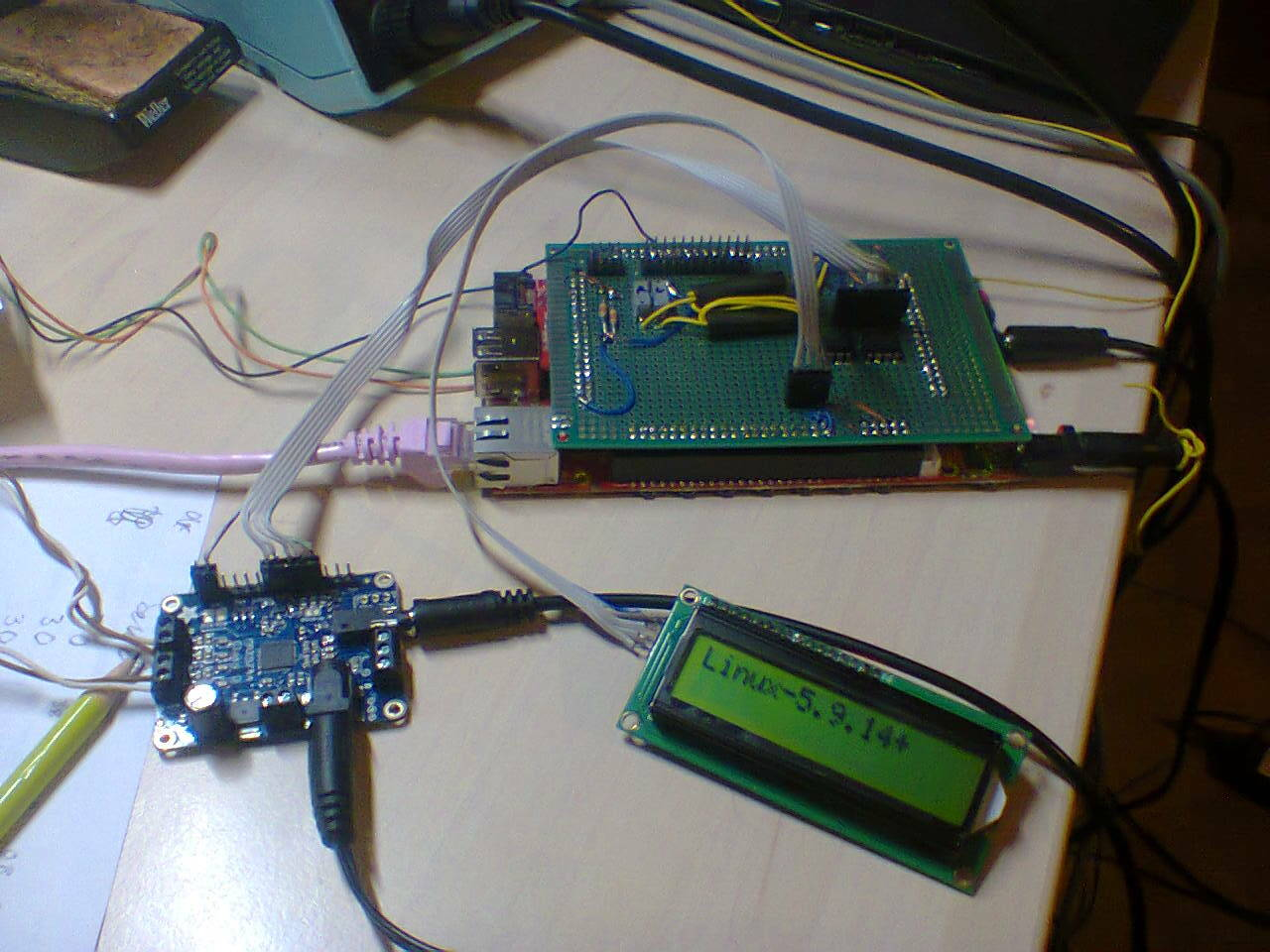For a project I'm using a text display containing the Hitachi HD44780 chip. These displays come in different sizes, common are 2 lines with 16 characters or 4 lines with 20 characters. The latter is also sold under the name 2004a. These displays use 5V. So these days with most CPUs and microcontrollers running with 3.3V or lower, the display is often connected to an I²C bus via a GPIO extender based on the PCF8574. The GPIO extenders are usually soldered to the display connector. You can buy the display and the GPIO extender separately or packaged together. You will always have to solder the GPIO extender to the display.

Now the correct way to connect the display to a 3.3V I²C-bus would be with a level-converter. But there is a hardware-hack to remove the pullup resistors on the PCF8574 breakout board (they're connected to +5V) which makes the device compatible with 3.3V installations: The minimum high logic level tolerated by the PCF8574 is given as 0.7 * VCC (which would mean 3.5V) but works in practice when driven with 3.3V. Note that the numbers of the resistors (R5/R6 in the hardware-hack link) may vary in different PCF8574 boards, I've seen R8, R9 for these resistors as well as no number at all. The resistors are 4.7 kΩ, usually labelled 472 in the SMD variant.

I investigated if there is a Linux driver for these displays and discovered one in drivers/auxdisplay/hd44780.c. On first glance the driver does not support I²C via the PCF8574 I/O expander. So I wrote a driver and submitted it to the kernel.

In the discussion (thanks, Geert) it turned out that there is a driver for the PCF8574 in the kernel (I had discovered so much) and that the HD44780 driver in the kernel can can be configured via appropriate device tree magic to use the I/O expander. The following device tree incantations define an overlay that configures the PCF8574 on its default I²C address 0x27 and then uses the I/O expander for configuring the I/Os for the hd44780 driver:

// Note that on most boards another fragment must enable the I2C-1 Bus

/dts-v1/;
/plugin/;

/ {
fragment@0 {
target = <&i2c1>;
__overlay__ {
#size-cells = <0>;

pcf8574: pcf8574@27 {
compatible = "nxp,pcf8574";
reg = <0x27>;
gpio-controller;
#gpio-cells = <2>;
};

};
};

fragment@1 {
target-path = "/";
__overlay__ {
hd44780 {
compatible = "hit,hd44780";
display-height-chars = <2>;
display-width-chars  = <16>;
data-gpios = <&pcf8574 4 0>,
<&pcf8574 5 0>,
<&pcf8574 6 0>,
<&pcf8574 7 0>;
enable-gpios = <&pcf8574 2 0>;
rs-gpios = <&pcf8574 0 0>;
rw-gpios = <&pcf8574 1 0>;
backlight-gpios = <&pcf8574 3 0>;
};
};
};
};

Since this is non-obvious not just to me (in my research I've discovered at least two out-of-tree Linux driver implementations of drivers for the HD44780 with the PCF8574 I/O expander) I've submitted a documentation patch to make this better documented for others searching a Linux driver.

Note that the driver for this display uses escape sequences to access the various special functions of the display (e.g. turning the backlight on and off, clearing the screen or defining user-defined character bitmaps). I think those are documented only in the source-code in drivers/auxdisplay/charlcd.c.